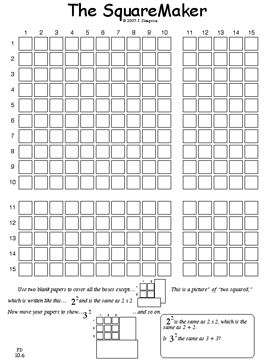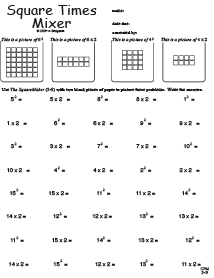K-8 Guided Student Discovery Math for small group, whole class, homeschool, and tutoring
 Our Books Multiplying and Dividing * The Best Stuff for Multiplying & Dividing * Teams * Discover the Basics - Multiplying & Dividing Adding and Subtracting * Addition, Subtraction, & Place Value Discover the Basics - Adding & Subtracting Fractions and Decimals * Fractions & Decimals Percents, Ratios, and Rates * Percents, Ratios, & Rates Access to Algebra * Discover the Basics - Equations! * Discover the Basics - Negative Numbers * Discover the Basics - Squares, Roots, & Exponents *Access to Algebra Organizing and Graphing Data * Organizing & Graphing Data Measurement and Geometry * Measurement & Geometry * Discover the Basics - Perimeter, Area, & Volume

 How it Works Discover and Perfect the power of Guided Student Discovery here: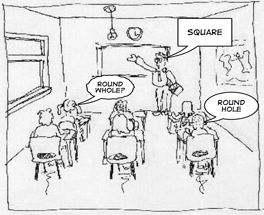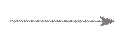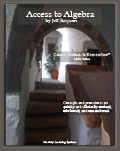Here are a few sample pages from the book.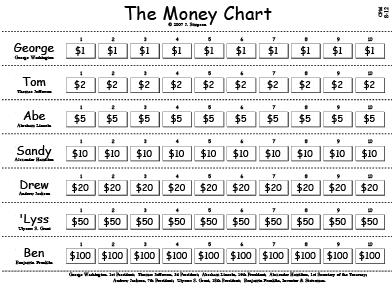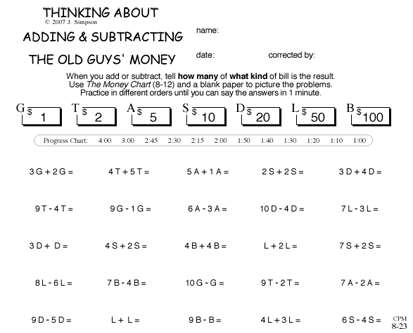________________________________________________Watch our Podcast, "The SquareMaker" to see how the MLS SquareMaker enables students to visualize, discover, and build a context derived understanding and memory of squares and roots.